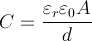## Dielectric materials

Dielectric materials are essentially insulators, which means that no current will flow through the material when a voltage is applied. However, certain changes do happen at the atomic scale. When a voltage is applied across a dielectric object, it becomes polarized. Since atoms are made of a positively charged nucleus and negatively charged electrons, polarization is an effect which slightly shifts electrons towards the positive voltage. They do not travel far enough to create a current flow through the material – the shift is microscopic, but has a very important effect, especially when dealing with capacitors. Once the voltage source is removed from the material, it either returns to its original non-polarized state, or stays polarized if the molecular bonds in the material are weak. The difference between the terms dielectric and insulator is not very well defined. All dielectric materials are insulators, but a good dielectric is one which is easily polarized.

The amount of polarization which occurs when a certain voltage is applied to an object influences the amount of electrical energy that is stored in the electric field. This is described by the dielectric constant of the material. The dielectric constant is not the only property of dielectric materials. Other properties such as dielectric strength and dielectric loss are equally important in the choice of materials for a capacitor in a given application.

### Dielectric constant

The dielectric constant of a material, also called the permittivity of a material, represents the ability of a material to concentrate electrostatic lines of flux. In more practical terms, it represents the ability of a material to store electrical energy in the presence of an electric field. All materials, including vacuum, store energy when placed in an electric field. The permittivity of vacuum is defined as the physical constant ε0, which is approximately ε0 = 8.854 x 10-12 farads per meter. This constant appears in many electromagnetism formulas.

Since most capacitors are not made of vacuum, it makes sense to define permittivity for every material. The permittivity of a material is defined as ε=εrε0, where ε is the absolute permittivity and er is the relative permittivity. εr is a number which is always greater than 1, meaning that all materials store more energy than free space when subjected to an electric field. This property is quite useful in capacitor applications, and we will explain this further in this article. It should be noted that the relative permittivity depends on many factors, such as temperature, pressure and even frequency, which is why materials with more stable dielectric constant are favored in some applications.

Different materials have different values of relative permittivity. Here we provide a list of materials commonly used in capacitors, along with their er values at the frequency of 1kHz at room temperature, which can be used as a quick reference and shows the wide range of values encountered in practice:

 Material εr Vacuum 1 Water 30-88 (depending on temperature) Glass 3.7-10 PTFE (Teflon) 2.1 Polyethylene (PE) 2.25 Polyimide 3.4 Polypropylene 2.2-2.36 Polystyrene 2.4-2.7 Titanium dioxide 86-173 Strontium titanate 310 Barium strontium titanate 500 Barium titanate 1250 – 10,000 (depending on temperature) Conjugated polymers 1.8 up to 100,000 (depending on type) Calcium copper titanate >250,000

### Dielectric strength

Unfortunately, there is a limit on the voltage an insulator can withstand before conducting electricity. All materials have an upper voltage limit, called breakdown voltage. A good example of this is air. It is considered an insulator, but under certain circumstances it can flow current. This is exactly what happens during a lightning strike. After the breakdown field strength is exceeded, air is ionized (electrons are torn away from the atom’s nucleus) and they begin moving under the influence of the electric field, producing electrical current. It is very important not to exceed the maximum rated voltage of a capacitor in order to prevent damage or even complete destruction. The dielectric strength for air is approximately 3 megavolts per meter. In comparison, the dielectric strength for mica is approximately 120 MV/m. The choice of dielectric material is very important in some applications where high voltages are expected, or when the thickness of the dielectric is very small.

### Dielectric loss

The term dielectric loss refers to the energy that is lost to heating of an object that is made of a dielectric material if a variable voltage is applied to it. These losses happen because as the material changes polarization, the tiny electron shifts can be regarded as a tiny alternating current flow. Different materials have different losses at different frequencies, and this characteristic must be accounted for in some high frequency applications.

## Application of dielectric materials to capacitors

In order to understand the effect of the dielectric on a capacitor, let us first quickly review the known formula for the capacitance of a parallel-plate capacitor:where C is the capacitance, εr is the relative permittivity of the material, ε0 is the permittivity of vacuum, A is the area of the plates and d is the distance between the plates. It becomes clear that the larger εr is, the larger the resulting capacitance becomes. For example, air as a material has a relative permittivity of approximately 1, meaning that it acts as if the capacitor plates were placed in a vacuum. On the other hand, some polymers can have the relative permittivity of up to 100,000! Using such materials, it is possible to achieve the same capacitance in a much smaller volume, opening possibility for miniaturization.

Now let’s look at the dielectric strength. Consider an air capacitor, with the distance between the electrodes being 0.1mm. The dielectric strength of air is 3 megavolts per meter. This means that the maximum voltage that can be applied to this example capacitor is 300 volts under ideal conditions. The smaller the capacitor, the lower the maximum allowed voltage. All capacitors have maximum rated voltages which depend on the materials used, and exceeding these rated values could damage or destroy the capacitor.